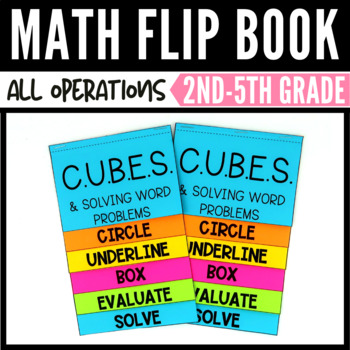# CUBES Math Strategy Flip BookSubject
Resource Type
Format
PDF (2 MB)
Standards
\$4.00
\$4.00

#### Also included in

1. The task cards in this bundle include concepts under the operations and algebraic thinking standards. The standards include; understanding division as an unknown factor, multiplication and division within 100, two-step word problems, and finding patterns in a multiplication chart. The unique set of
\$18.00
\$24.00
Save \$6.00
2. The bundle includes flip books that cover the strategies of multiplication, properties of multiplication, and the CUBES math strategy for word problems. Each flip book provides a reference for your math students that they can store in their interactive notebooks.See the preview to view pictures of e
\$15.00
\$20.00
Save \$5.00
3. This is not your average multiplication and division task card bundle. The resource also comes with and interactive notebook option with interactive notebook pockets and flip books for each volume. You can use the task cards in math centers, math rotations, small groups, for bell-ringers, etc... The
\$49.00
\$84.50
Save \$35.50
4. Whether you are trying to reinforce addition fluency, subtraction fluency, and word problem strategies in your classroom or strengthing student knowledge at home with homeschool or distance learning, these flip books are perfect.What's included:Addition Flip Book (Facts 1-10/Addition to 20)Help your
\$9.00
\$12.00
Save \$3.00

### Description

This CUBES math strategy flip book helps students with those tough math word problems. The flip book provides your students with tips to break down intimidating word problems and solve them with success.

Plus, these flip books fit perfectly in interactive math notebooks!

You may also like these flip books:

Multiplication Flip Book

Properties of Multiplication Flip Book 3rd Grade

For typos please email me at mracruz@hotmail.com with the subject stating which resource you are requesting a typo fix.

LET'S STAY CONNECTED!

Total Pages
N/A
N/A
Teaching Duration
N/A
Report this Resource to TpT
Reported resources will be reviewed by our team. Report this resource to let us know if this resource violates TpT’s content guidelines.

### Standards

to see state-specific standards (only available in the US).
Solve multistep word problems posed with whole numbers and having whole-number answers using the four operations, including problems in which remainders must be interpreted. Represent these problems using equations with a letter standing for the unknown quantity. Assess the reasonableness of answers using mental computation and estimation strategies including rounding.
Multiply or divide to solve word problems involving multiplicative comparison, e.g., by using drawings and equations with a symbol for the unknown number to represent the problem, distinguishing multiplicative comparison from additive comparison.
Use multiplication and division within 100 to solve word problems in situations involving equal groups, arrays, and measurement quantities, e.g., by using drawings and equations with a symbol for the unknown number to represent the problem.
Use addition and subtraction within 100 to solve one- and two-step word problems involving situations of adding to, taking from, putting together, taking apart, and comparing, with unknowns in all positions, e.g., by using drawings and equations with a symbol for the unknown number to represent the problem.
Solve word problems that call for addition of three whole numbers whose sum is less than or equal to 20, e.g., by using objects, drawings, and equations with a symbol for the unknown number to represent the problem.